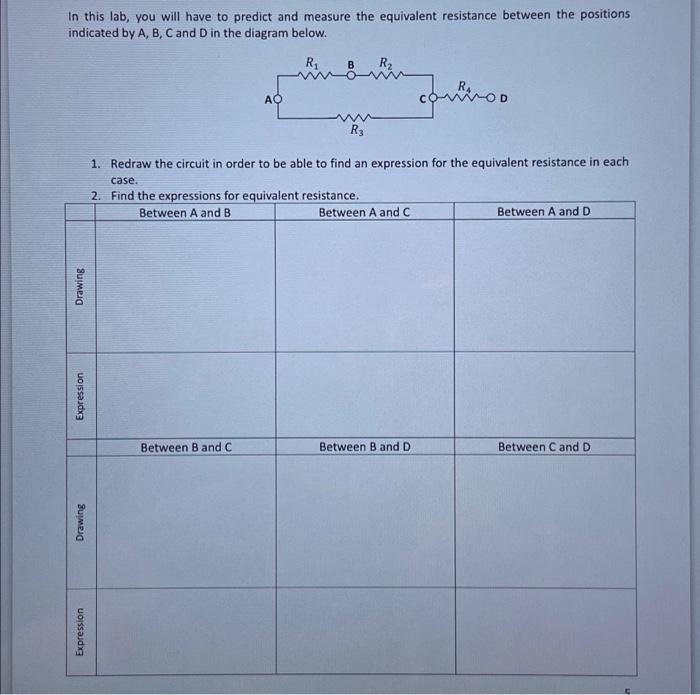# Question Solved1 Answerquestion 1 &2 pls In this lab, you will have to predict and measure the equivalent resistance between the positions indicated by A, B, C and D in the diagram below. R B R2 АО connoo w R3 1. Redraw the circuit in order to be able to find an expression for the equivalent resistance in each case. 2. Find the expressions for equivalent resistance. Between A and B Between A and C Between A and D Drawing Expression Between Band C Between B and D Between C and D Drawing Expressionquestion 1 &2 plsTranscribed Image Text: In this lab, you will have to predict and measure the equivalent resistance between the positions indicated by A, B, C and D in the diagram below. R B R2 АО connoo w R3 1. Redraw the circuit in order to be able to find an expression for the equivalent resistance in each case. 2. Find the expressions for equivalent resistance. Between A and B Between A and C Between A and D Drawing Expression Between Band C Between B and D Between C and D Drawing Expression
More
Transcribed Image Text: In this lab, you will have to predict and measure the equivalent resistance between the positions indicated by A, B, C and D in the diagram below. R B R2 АО connoo w R3 1. Redraw the circuit in order to be able to find an expression for the equivalent resistance in each case. 2. Find the expressions for equivalent resistance. Between A and B Between A and C Between A and D Drawing Expression Between Band C Between B and D Between C and D Drawing Expression